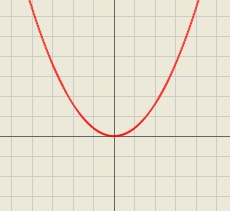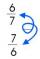# Reciprocal equation 2

Solve this equation:

x + 5/x - 6 = 4/11

x1 =  5.4454
x2 =  0.9182

### Step-by-step explanation:Did you find an error or inaccuracy? Feel free to write us. Thank you!Tips to related online calculators
Looking for help with calculating roots of a quadratic equation?
Do you have a linear equation or system of equations and looking for its solution? Or do you have a quadratic equation?

## Related math problems and questions:

• Reciprocal equation 3Solve reciprocal equation: 1/2 + 2/3=1/x
• Eq with reciprocalSolve given equation with reciprocal member: a-6/a+10=4/8
• Eq1Solve equation: 4(a-3)=3(2a-5)
• Sequence 11What is the nth term of this sequence 1, 1/2, 1/3, 1/4, 1/5?
• Equation 25Solve following simple equation: 3/4(x+5)=1/2(x+9)
• Simply equationSolve this equation for x: 9x-6(x-1)=5(x+2)-11
• Fraction + eqSolve following simple equation with fractions: -5/6(8+5b) = 75 + 5/3b
• Simple equationSolve the following simple equation: 2. (4x + 3) = 2-5. (1-x)Add marks (+, -, *, /, brackets) to fullfill equations 1 3 6 5 = 10 This is for the 4th grade of the primary school - with no negative numbers yet
• Eq2 2Solve following equation with quadratic members and rational function: (x2+1)/(x-4) + (x2-1)/(x+3) = 23
• EquatiomSolve equation with negatives: X/(-5) + 2 = -9
• Expression 8Evaluate this expressions: a) 5[3 + 4(2.8 - 3)] b) 5×(8-4)÷4-2
• EquationSolve equation and check the result: 1.4x - 3/2 + x - 9,8 = x + 0,4/3 - 7 + 1,6/6
• ReciprocalCalculate the reciprocal numbers for the given real numbers.Why does 1 3/4 + 2 9/10 equal 4.65? How do you solve this?(a) Convert the following mixed numbers to improper fractions. i. 3 5/8 ii. 7 7/6 (b) Convert the following improper fraction to mixed number. i. 13/4 ii. 78/5 (c) Simplify these fractions to their lowest terms. i. 36/42 ii. 27/45 2. evaluate following exSolve equation with fractions: X × 3/8 = 1/2